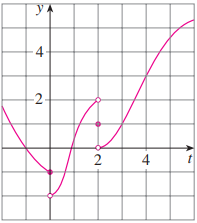×

×

# For the function t whose graph is given, state the value of each quantity, if it existsISBN: 9781305270336 484

## Solution for problem 7 Chapter 2.2

Single Variable Calculus: Early Transcendentals | 8th Edition

• Textbook Solutions
• 2901 Step-by-step solutions solved by professors and subject experts
• Get 24/7 help from StudySoup virtual teaching assistantsSingle Variable Calculus: Early Transcendentals | 8th Edition

4 5 1 366 Reviews
30
2
Problem 7

For the function t whose graph is given, state the value of each quantity, if it exists. If it does not exist, explain why. (a) lim tl02 tstd (b) lim tl01 tstd (c) lim tl0 tstd (d) lim tl22 tstd (e) lim tl21 tstd (f) lim tl2 tstd (g) ts2d (h) lim tl4 tstd

Step-by-Step Solution:

Problem 7

For the function t whose graph is given, state the value of each quantity, if it exists. If it does not exist, explain why

(a)(b)(c)(d)(e)(f)(g) g (2)

(h)Step by Step Solution

Step 1 of 8

The given graph of the function g is(a) To find. From the graph, it is seen that as t approaches to 0 from its left side, g(t) approaches to -1. Therefore,Step 2 of 8

Step 3 of 8

##### ISBN: 9781305270336

The answer to “For the function t whose graph is given, state the value of each quantity, if it exists. If it does not exist, explain why. (a) lim tl02 tstd (b) lim tl01 tstd (c) lim tl0 tstd (d) lim tl22 tstd (e) lim tl21 tstd (f) lim tl2 tstd (g) ts2d (h) lim tl4 tstd” is broken down into a number of easy to follow steps, and 54 words. This full solution covers the following key subjects: . This expansive textbook survival guide covers 95 chapters, and 5427 solutions. Since the solution to 7 from 2.2 chapter was answered, more than 228 students have viewed the full step-by-step answer. This textbook survival guide was created for the textbook: Single Variable Calculus: Early Transcendentals, edition: 8. The full step-by-step solution to problem: 7 from chapter: 2.2 was answered by , our top Calculus solution expert on 03/19/18, 03:29PM. Single Variable Calculus: Early Transcendentals was written by and is associated to the ISBN: 9781305270336.

Unlock Textbook Solution